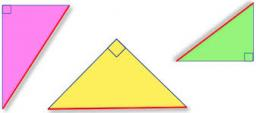# Hypotenuse 3554

Calculate the hypotenuse length if you know the area of an isosceles right triangle that is 24.5 cm square.

c =  9.8995 cm

### Step-by-step explanation:

Try calculation via our triangle calculator.Did you find an error or inaccuracy? Feel free to write us. Thank you!

Tips for related online calculators
The Pythagorean theorem is the base for the right triangle calculator.